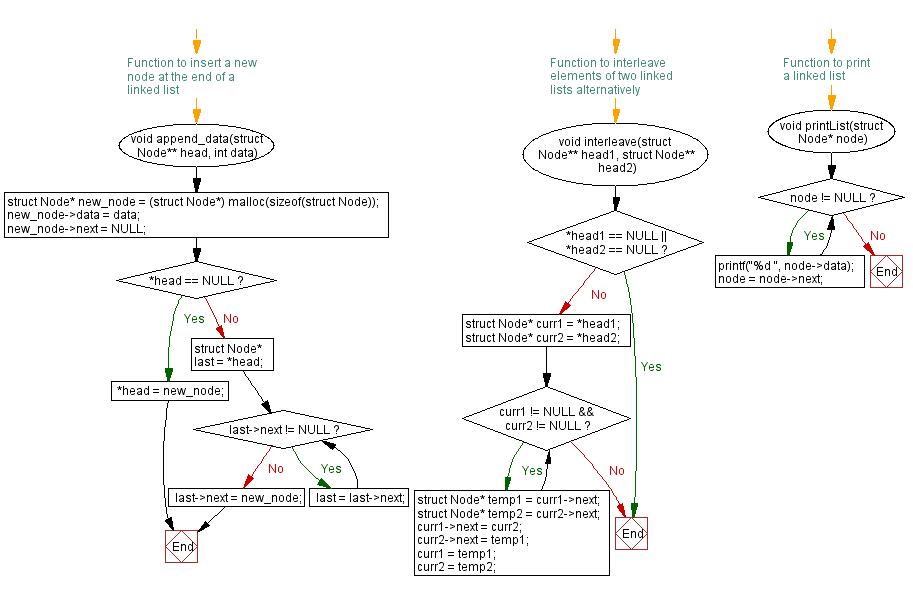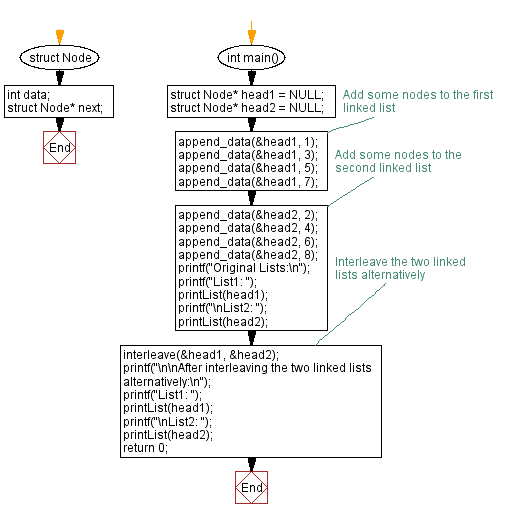﻿ C Program: Interleave elements of two linked lists alternatively

# C Exercises: Interleave elements of two linked lists alternatively

## C Singly Linked List : Exercise-39 with Solution

Write a C program to interleave elements of two singly linked lists alternatively.

Sample Solution:

C Code:

``````#include<stdio.h>
#include <stdlib.h>

struct Node {
int data;
struct Node* next;
};

// Function to insert a new node at the end of a linked list
void append_data(struct Node** head, int data) {
struct Node* new_node = (struct Node*) malloc(sizeof(struct Node));
new_node->data = data;
new_node->next = NULL;

} else {
while (last->next != NULL) {
last = last->next;
}
last->next = new_node;
}
}

// Function to interleave elements of two linked lists alternatively
return;
}

while (curr1 != NULL && curr2 != NULL) {
struct Node* temp1 = curr1->next;
struct Node* temp2 = curr2->next;

curr1->next = curr2;
curr2->next = temp1;

curr1 = temp1;
curr2 = temp2;
}
}

// Function to print a linked list
void printList(struct Node* node) {
while (node != NULL) {
printf("%d ", node->data);
node = node->next;
}
}
int main() {

printf("Original Lists:\n");
printf("List1: ");
printf("\nList2: ");

// Interleave the two linked lists alternatively
printf("\n\nAfter interleaving the two linked lists alternatively:\n");
printf("List1: ");
printf("\nList2: ");
return 0;
}
```
```

Sample Output:

```Original Lists:
List1: 1 3 5 7
List2: 2 4 6 8

After interleaving the two linked lists alternatively:
List1: 1 2 3 4 5 6 7 8
List2: 2 3 4 5 6 7 8
```

Flowchart :C Programming Code Editor:

What is the difficulty level of this exercise?

﻿